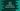# Java program to check if a Matrix is Sparse Matrix or Dense Matrix## What is a sparse matrix ?

A sparse matrix is a matrix in which most of the values are zero. Similarly a matrix with maximum non-zero values is known as dense matrix. In this tutorial, we will learn how to check if a matrix is sparse or not in java.

### Sparsity of a matrix :

The proportion of zero elements to non-zero elements of a matrix is called sparsity. If a matrix contains 9 zero values and 3 non-zero values, the sparsity will be 9/3 = 3 .

### Checking a matrix sparse or not programatically :

To check if a matrix is sparse-matrix or not, we will count the number of zeros in the matrix. If the count is more than half of the numbers, it will be a sparse-matrix and otherwise a Dense matrix. For a matrix with ‘r’ no of rows and ‘c’ no of columns, the total number of elements will be ‘r*c’ .

Let’s take a look into the java program to find if a matrix is sparse or Dense :

``````import java.util.Scanner;

public class Example {

public static void main(String[] args) {
int row = 0;
int col = 0;

int count = 0; //number of zero counts

Scanner sc = new Scanner(System.in);

System.out.println("Please enter the row numbers for the matrix : ");
row = sc.nextInt();

System.out.println("Please enter the column numbers for the matrix : ");
col = sc.nextInt();

int[][] matrix = new int[row][col];

for (int i = 0; i < row; i++) {
System.out.println("Please enter row no : " + (i + 1));
for (int j = 0; j < col; j++) {
matrix[i][j] = sc.nextInt();
if (matrix[i][j] == 0) {
count++;
}
}
}
if (count > (row * col) / 2) {
System.out.println("The above matrix is a Sparse matrix ");
} else {
System.out.println("The above matrix is a Dense matrix");
}

}
}``````

### Sample Output :

``````Please enter the row numbers for the matrix :
3
Please enter the column numbers for the matrix :
3
Please enter row no : 1
1 0 0
Please enter row no : 2
2 0 0
Please enter row no : 3
0 0 3
The above matrix is a Sparse matrix``````